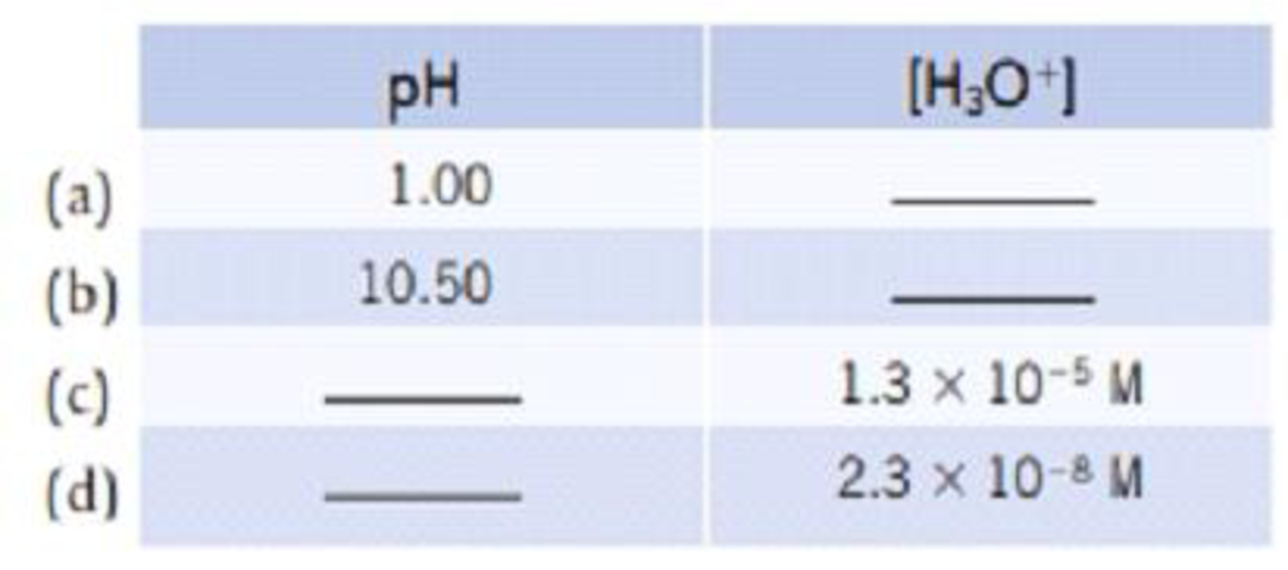# Make the following conversions. In each case, tell whether the solution is acidic or basic.### Chemistry & Chemical Reactivity

9th Edition
John C. Kotz + 3 others
Publisher: Cengage Learning
ISBN: 9781133949640

#### Solutions

Chapter
Section### Chemistry & Chemical Reactivity

9th Edition
John C. Kotz + 3 others
Publisher: Cengage Learning
ISBN: 9781133949640
Chapter 4, Problem 59PS
Textbook Problem
1 views

## Make the following conversions. In each case, tell whether the solution is acidic or basic.(a)

Interpretation Introduction

Interpretation:

The pH of 1.00 has to be converted into [H3O+] and whether it is acidic or basic has to be determined.

Concept introduction:

• pH of a solution is the negative of the base -10 logarithm of the hydronium ion concentration.

pH=-log[H3O+]

• Concentration of hydronium ion [H3O+]=10-pH
• For an acidic solution pH<7 and for a basic solution pH>7.

### Explanation of Solution

The given solution has a pH of 1.00 and its hydronium ion concentration can be determined by using the equation,

[H3O+]=10-pH

Substituting pH value of solution a in the above equation,

[H3O+]=10-(1

(b)

Interpretation Introduction

Interpretation:

The pH of 10.50 has to be converted into [H3O+] and whether it is acidic or basic has to be determined.

Concept introduction:

• pH of a solution is the negative of the base -10 logarithm of the hydronium ion concentration.

pH=-log[H3O+]

• Concentration of hydronium ion [H3O+]=10-pH
• For an acidic solution pH<7 and for a basic solution pH>7.

(c)

Interpretation Introduction

Interpretation:

The [H3O+] has to be converted into pH and whether it is acidic or basic has to be determined.

Concept introduction:

• pH of a solution is the negative of the base -10 logarithm of the hydronium ion concentration.

pH=-log[H3O+]

• Concentration of hydronium ion [H3O+]=10-pH
• For an acidic solution pH<7 and for a basic solution pH>7.

(d)

Interpretation Introduction

Interpretation:

The [H3O+] has to be converted into pH and whether it is acidic or basic has to be determined.

Concept introduction:

• pH of a solution is the negative of the base -10 logarithm of the hydronium ion concentration.

pH=-log[H3O+]

• Concentration of hydronium ion [H3O+]=10-pH
• For an acidic solution pH<7 and for a basic solution pH>7.

### Still sussing out bartleby?

Check out a sample textbook solution.

See a sample solution

#### The Solution to Your Study Problems

Bartleby provides explanations to thousands of textbook problems written by our experts, many with advanced degrees!

Get Started

Find more solutions based on key concepts
Most codons, specify a(n) ___ . a. protein b. polypeptide c. amino acid d. mRNA

Biology: The Unity and Diversity of Life (MindTap Course List)

Blood LDL values of people eating typical, meat-rich Western diets are generally higher than LDL values of vege...

Nutrition: Concepts and Controversies - Standalone book (MindTap Course List)

Match each substance with its correct description

Human Biology (MindTap Course List)

A uniform beam of length 7.60 m and weight 4.50 102 N is carried by two workers, Sam and Joe, as shown in Figu...

Physics for Scientists and Engineers, Technology Update (No access codes included)

Where is the oxygen on Mars today? How do you know?

Foundations of Astronomy (MindTap Course List)

How does sand move on a beach?

Oceanography: An Invitation To Marine Science, Loose-leaf Versin